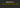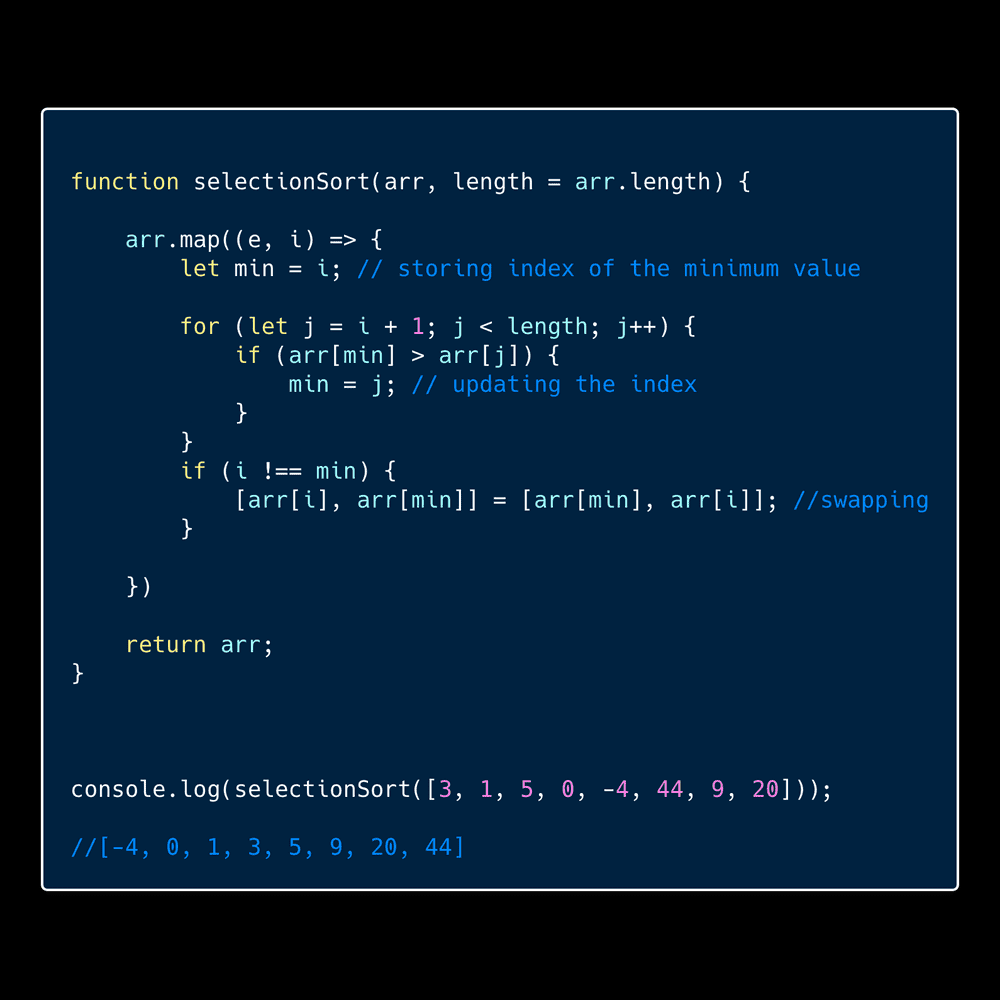# How to implement Selection sort algorithm in JavaScriptIn this tutorial, we will learn about selection sort algorithm and its implementation in JavaScript.

## What is Selection sort?

Selection sort is a sorting algorithm with O(n2) time complexity.It performs very slow on larger datasets.

## How does selection sort works?

Selection sort starts with choosing a minimum element and compares it against the unsorted array.

Example:

`` [ 4,3,5,2 ]``

selection sort chooses 4 as the minimum element and starts comparing it against the remaining part of the array if any element which is smaller than 4 then we need to swap it.

``[ 2,3,5,4]``

After swapping now it chooses 3 as a minimum element and starts comparing with remaining part of the array there is no element which is smaller than 3 so we move to next element which is 5.

Now 5 is a minimum element but we find the element 4 which is smaller than 5 so that we swap it.

final sorted array `[ 2,3,4,5 ]`.

### Algorithm implementation

So far you have got some knowledge about how selection sort works, so Let’s implement the selection sort algorithm now.

``````function selectionSort(arr){
return arr;
}``````

Now, we need to use two for loops one is for choosing the minimum element and the second one is comparing it against the remaining part of the unsorted array and updates the minimum element.

``````function selectionSort(arr) {

for (var i = 0; i < arr.length; i++) {

let min = i; //  storing the index of minimum element

for (var j = i + 1; j < arr.length; j++) {
if (arr[min] > arr[j]) {
min = j; // updating the index of minimum element
}
}
}
return arr;
}``````

The final step is we need to swap.

``````function selectionSort(arr) {

for (var i = 0; i < arr.length; i++) {

let min = i; //  storing the index of minimum element

for (var j = i + 1; j < arr.length; j++) {
if (arr[min] > arr[ j ]) {
min = j; // updating the index of minimum element
}
}

if (i !== min) {
let temp = arr[ i ];            arr[ i ] = arr[min];            arr[min] = temp;        }
}
return arr;
}``````

Similarly, you can even use the array destructuring for swapping the elements.

``````function selectionSort(arr) {

for (var i = 0; i < arr.length; i++) {

let min = i; //  storing the index of minimum element

for (var j = i + 1; j < arr.length; j++) {
if (arr[min] > arr[j]) {
min = j; // updating the index of minimum element
}
}

if (i !== min) {
[arr[ i ],arr[min]]= [arr[min],arr[ i ]];        }
}
return arr
}

console.log(selectionSort([ 4,3,5,2 ])) // [2,3,4,5]``````

### Second way of implementation using the JavaScript map method## Top Udemy Courses##### JavaScript - The Complete Guide 2020 (Beginner + Advanced)
45,614 students enrolled
52 hours of video content
View Course##### React - The Complete Guide (incl Hooks, React Router, Redux)
284,472 students enrolled
40 hours of video content
View Course##### Vue JS 2 - The Complete Guide (incl. Vue Router & Vuex)
130,921 students enrolled
21 hours of video content
View Course